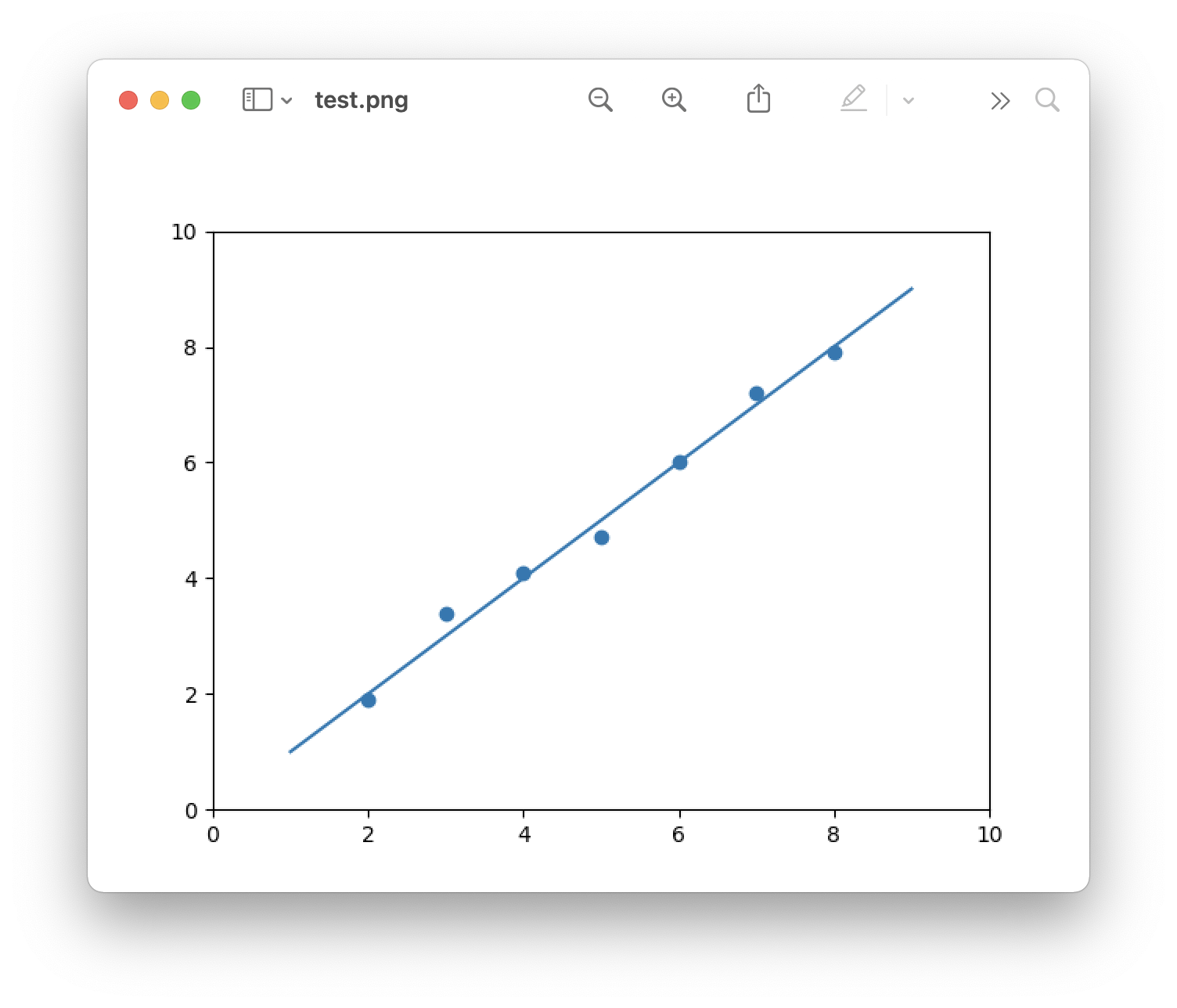The matplotlib 'show' function opens a window with a diagram. Did you know you can also render the plot and save as an image?

# Save a matplotlib diagram as a PNG image.

For a test project, I need to test a lot of different values in a diagram. To prevent having to close the window that is created by matplotlib each time before testing new values, I render the plot to an image and switch to the open image in macOS preview.

This is what the result looks like:In order for it to work, first pip-install matplotlib.

`pip install matplotlib`

And here is the code to save the plot as a PNG file.

```import matplotlib.pyplot as plt

fig, ax = plt.subplots()
plt.xlim(0, 10)
plt.ylim(0, 10)

x = [2, 3, 4, 5, 6, 7, 8]
y = [1.9, 3.4, 4.1, 4.7, 6, 7.2, 7.9]

sc = ax.scatter(x, y)
plt.plot([1, 9], [1, 9])
plt.savefig('test.png')```Written by Loek van den Ouweland on 2021-08-17.
Questions regarding this artice? You can send them to the address below.